0
8193

# RRB NTPC Reasoning Questions PDF

Download RRB NTPC Reasoning Questions and Answers PDF. Top 25 RRB NTPC  Maths  questions based on asked questions in previous exam papers very important for the Railway NTPC exam.Question 1: A standard dice is as shown. The sum of the numbers on the opposite faces of a standard dice is always the same irrespective of which faces are chosen. What is the number on the bottom face?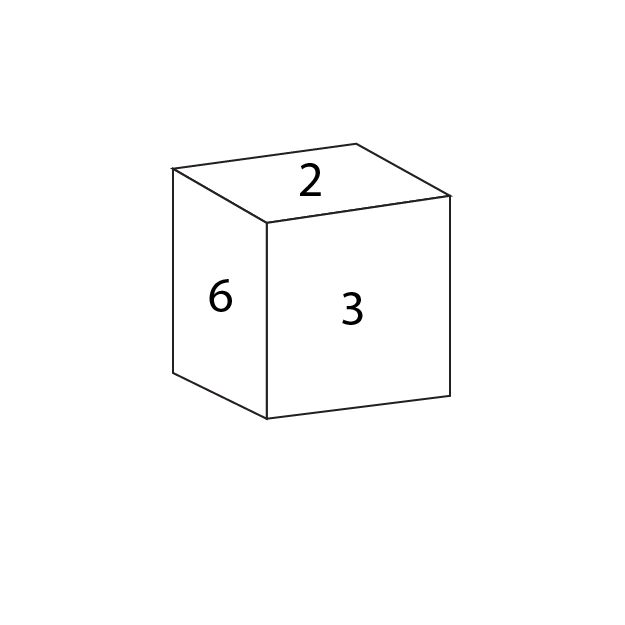a) 1

b) 4

c) 5

d) Can’t say

Question 2: Find the missing number?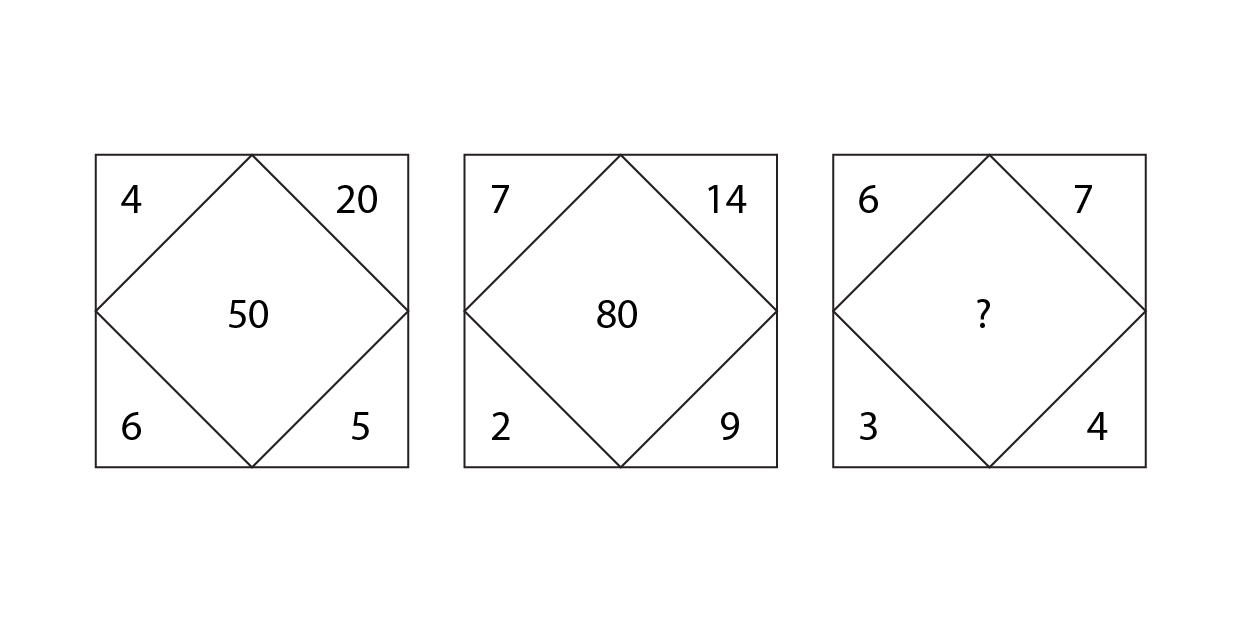a) 30

b) 40

c) 50

d) 70Question 3: Find the missing number?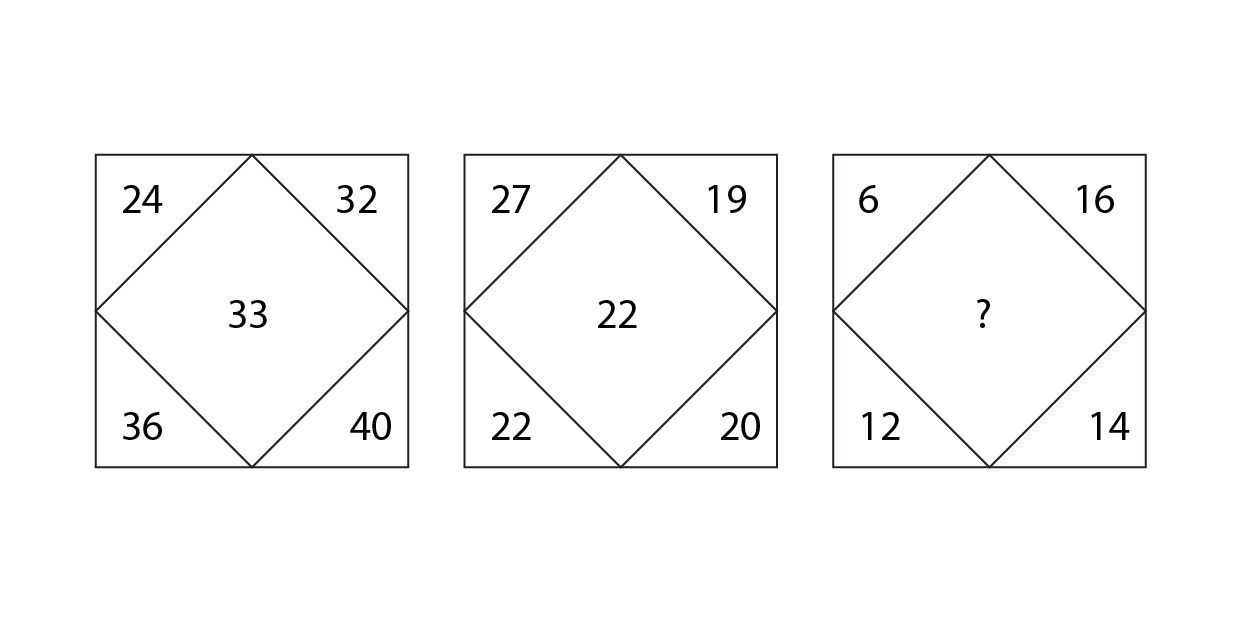a) 10

b) 12

c) 14

d) 11

Question 4: How many triangles do you see?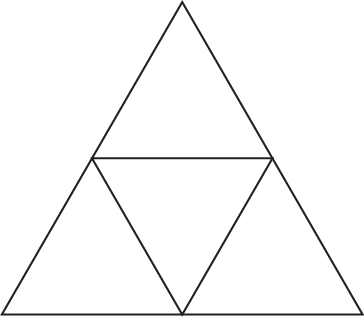a) 3

b) 4

c) 5

d) More than 5

Question 5: How many squares are there in this figure?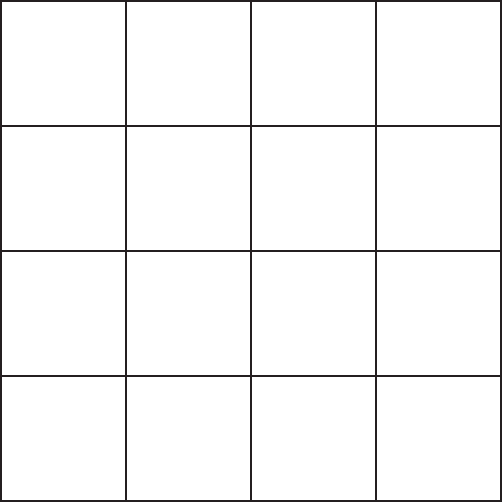a) 16

b) 27

c) 29

d) 30Question 6: Study the figures shown in the options below. Three of them are alike in a certain way and hence form a group. Identify the one that doesn’t belong to that group?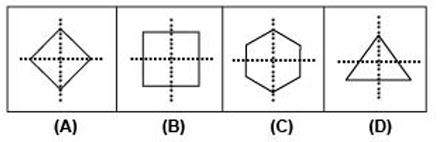a) A

b) B

c) C

d) D

Question 7: Three of the four figures given in the options are alike in a certain way and hence form a group. Which of the following doesn’t belong to that group?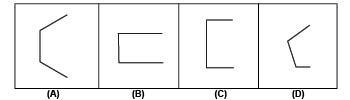a) A

b) B

c) C

d) D

Question 8: Three of the four figures given in the options are alike in a certain way and hence form a group. Select the option that does not belong to the group.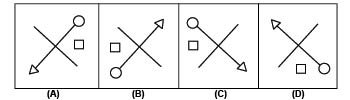a) A

b) B

c) C

d) D

Question 9: Three of the four figures are alike in a certain way and hence form a group. Which of the following figures doesn’t belong to that group?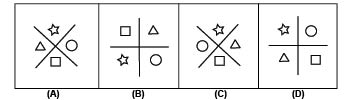a) A

b) B

c) C

d) D

Question 10: Three of the four figures are alike in a certain way and hence form a group. Which of the following figures doesn’t belong to that group?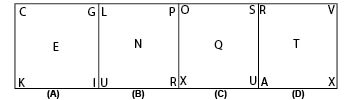a) A

b) B

c) C

d) D

Question 11: Three of the four figures are alike in a certain way and hence form a group. Which of the following doesn’t belong to the group?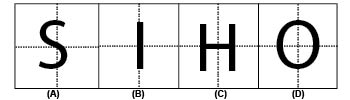a) A

b) B

c) C

d) D

Question 12: Find the number which must be opposite to 6 on the given dice.

a) 5

b) 4

c) 1

d) Can’t be said with certainty

Question 13: Find the number which will be opposite to 4 in the cubes shown below.

b) 2

c) 3

d) Cannot be determinedQuestion 14: Four figures are given below. Three of these figures can be grouped together while fourth cannot be put in the same group. Identify the odd one out.

a)

b)

c)

d)

Question 15: Pick the option which should come next in the series.

a)

b)

c)

d)

Question 16: Which figure among the option must logically replace the ‘?’ in the diagram below –

a)

b)

c)

d)

Question 17: A figure is given below. Choose the option in which the question figure is embedded.

a)

b)

c)

d)

Question 18: Which of the following images must logically complete the given series?

a)

b)

c)

d)

Question 19: Five friends Akhil, Brinda, Cyril, Dharani, and Edward are born in the same year. The months in which the friends are born are January, March, August, September, and December. Further, it is known that Akhil is not the youngest or the oldest among the friends. The same is the case with Dharani. Cyril is older than Brinda but younger than Edward. Cyril is older than Dharani.

Which of the following pairs (name and month of birth) is definitely matched incorrectly?

a) Cyril – August
b) Dharani – August
c) Akhil – March
d) Dharani – March

Question 20: Amar, Arun, Arjun, and Akhil are 4 friends working in Delhi. Each one of them is from a different city among Chennai, Bangalore, Hyderabad, and Pune. Further, it is known that,

Amar is not from Chennai or Bangalore.
Arjun is not from Hyderabad or Pune.
Akhil is not from Pune or Bangalore.
Arun is not from Chennai or Hyderabad.

Consider the following statements.

1.Amar is not from Pune.
2.Arjun is from Chennai.
3.Akhil is not from Bangalore

Each of the statements given above, when considered individually, results in a person-city distribution. The person city distribution obtained for which of the following statements is the same?

a) 1 and 2
b) 2 and 3
c) 1 and 3
d) All 3 statements result in the same distributionOpposite faces of a dice add up to 7. Hence, the bottom face=7-2=5.

The number in the center is the product of the top two numbers minus the product of the bottom two numbers. Hence, the missing number=42-12=30.

The number in the center is the average of all the numbers in the corners. Hence, the missing number = (6+16+12+14) /4= 48/4=12

There are 5 triangles – 4 small ones and one large triangle.

There are 16 squares of 1 unit. There are 9 squares of 4 units. There are 4 squares of 9 units and there is 1 square of 16 units. Hence, there are 16+9+4+1=30 squares.All the figures shown are symmetrical over the X axis except (d).

In the given options, we can observe that all the figures have regular polygons cut into half. (a) is half of a regular hexagon (b) is half of a rectangle (c) is half of a regular quadrilateral (square). (d) is an half of a pentagon, which is also a regular polygon.

In each of the options, the square is to the clockwise side of the circle except in (c), where the square is to the anti-clockwise side.

In each of the figures, the star is opposite to the square and the circle is opposite to the triangle, except (b).

In each of the following figures, the series starts with the top left letter.
For example, in (b), L is the first letter. The second letter is L+ 2 = N. The third letter is N + 2 = P. The fourth letter is P + 2 = R. The fifth letter is R + 3 = U.
So, this pattern is followed in every diagram except (a), which follows 2 + 2+ 2+ 2.

In the figures shown, all are symmetric on both the X axis and the Y axis except (a), which is not symmetric on either the X axis or the Y axis.

From the first two figures, we can infer that 1 must be opposite to 3. From the third figure we can see that 2 is opposite to 5. Hence, 4 must be opposite to 6. Thus, option B is the correct answer.

From the two given cubes, we can see that 2, 1, 5 and 6 are adjacent to 3. Hence, 4 must be opposite to 3. Thus, option C is the correct answer.

Options A, C and D are closed figures while option B is an open figure. Hence, option B is the odd one out.

The pattern being followed here is that in the second figure 3 addition lines are added to the first figure. In third figure 1 line is subtracted from the second figure. In the fourth figure 3 lines are again added. Hence, one line will be subtracted from the fourth figure to get the missing figure. Hence, option A is the correct answer.

We see that new symbol is added everytime at the start and the earlier symbols move along the sides of the square in clockwise direction. Thus we get,

Hence, option B is right choice.Option A is missing the square which is given in the question figure. Option B miss out on the vertical and horizontal lines at the center of the square. The question figure is embedded in the option D. Hence, option D is the correct answer.

The number of sides of the image at the top left corner increases by 1 with each subsequent step. Therefore, the answer image must contain a hexagon in the top left corner.

The circle alternates between being dark and light. Therefore, in the answer, the circle must be light.

The sector at the top right corner rotates 90 degrees in the clockwise direction. Therefore, in the answer, the incomplete part of the circle must be in the top left quadrant of the circle.

The horse shoe shaped object at the bottom right corner rotates 90 degrees in the clockwise direction. Therefore, the open end of the horse shoe must face the left edge in the answer.

The triple arrow setup at the centre of the image rotates 90 degrees anticlockwise in each subsequent step. Therefore, the unbalanced arrow must be facing the left edge in the answer image.

Only option A satisfies all these conditions. Therefore, option A is the right answer.

It has been given that neither Akhil nor Dharani is the oldest or youngest among the five persons. Therefore, they should be the second, third, or fourth oldest person in the group. Cyril is older than Brinda but younger than Edward. Therefore, Edward must be the oldest person and Brinda must be the youngest person in the group.

Cyril is older than Dharani. Therefore, 3 arrangements are possible.

Akhil, Cyril, Dharani
Cyril, Akhil, Dharani
Cyril, Dharani, Akhil

Edward – January
Brinda – December
Akhil – March, August, September
Dharani – August, September
Cyril – March, August
As we can see, Dharani should have born in either August or September and hence, option D is the right answer.